JEE  >  Mathematics Test 11 - Straight Line, Circle

# Mathematics Test 11 - Straight Line, Circle

Test Description

## 30 Questions MCQ Test JEE Main & Advanced Mock Test Series | Mathematics Test 11 - Straight Line, Circle

Mathematics Test 11 - Straight Line, Circle for JEE 2023 is part of JEE Main & Advanced Mock Test Series preparation. The Mathematics Test 11 - Straight Line, Circle questions and answers have been prepared according to the JEE exam syllabus.The Mathematics Test 11 - Straight Line, Circle MCQs are made for JEE 2023 Exam. Find important definitions, questions, notes, meanings, examples, exercises, MCQs and online tests for Mathematics Test 11 - Straight Line, Circle below.
Solutions of Mathematics Test 11 - Straight Line, Circle questions in English are available as part of our JEE Main & Advanced Mock Test Series for JEE & Mathematics Test 11 - Straight Line, Circle solutions in Hindi for JEE Main & Advanced Mock Test Series course. Download more important topics, notes, lectures and mock test series for JEE Exam by signing up for free. Attempt Mathematics Test 11 - Straight Line, Circle | 30 questions in 60 minutes | Mock test for JEE preparation | Free important questions MCQ to study JEE Main & Advanced Mock Test Series for JEE Exam | Download free PDF with solutions
 1 Crore+ students have signed up on EduRev. Have you?
Mathematics Test 11 - Straight Line, Circle - Question 1

### A point P on y-axis is equidistant from the points A(–5,4) and B(3,–2). Its co-ordinates are

Mathematics Test 11 - Straight Line, Circle - Question 2

### If the point P(x,y) be equidistant from the points A(a b, b–a) and B(a–b,a b), then

Detailed Solution for Mathematics Test 11 - Straight Line, Circle - Question 2
PA=PB
take square both side
PA^2=PB^2

now use distance
formula ,
{x-(a+b)}^2+{y-(b-a)}^2={x-(a-b)}^2+{y-(a+b)}^2

=>x^2+(a+b)^2-2x(a+b)+y^2+(b-a)^2-2y(b-a)y=x^2+(a-b)^2-2x(a-b)+y^2+(a+b)^2-2y(a+b)

=>2x(a-b)-2x(a+b)=2y(b-a)-2y(a+b)

=>2x{a-b-a-b}=2y{b-a-a-b}

=>2x(-2b)=2y(-2a)

=>bx=ay
Mathematics Test 11 - Straight Line, Circle - Question 3

### The points (0,8/3),(1,3) and (82,30) are the vertices of

Mathematics Test 11 - Straight Line, Circle - Question 4

P(3,1),Q(6,5) and R(x,y) are three points such that the angle PRQ is a right angle and the area of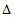RPQ= 7, then the number of such point R is

Mathematics Test 11 - Straight Line, Circle - Question 5

Determine the ratio in which the line 3x + 4y - 9 = 0 divides the line segment joining the points (1,3) and (2,7).

Mathematics Test 11 - Straight Line, Circle - Question 6
Given four lines with equations x+2y–3 = 0, 3x+4y–7 = 0, 2x+3y–4 = 0, 4x+5y–6 = 0, then
Mathematics Test 11 - Straight Line, Circle - Question 7

......PS be the median of the triangle with vertices P(2,2), Q(6,–1) and R(7,3). The equation of the line passing through (1,–1) and parallel to PS is

Mathematics Test 11 - Straight Line, Circle - Question 8
The line 4x+y =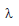cuts the axis of co-ordinates at A and B. If C is the foot of perpendicular drawn from origin O, then AC : CB =
Mathematics Test 11 - Straight Line, Circle - Question 9
Points on the line x+y = 4 that lie at a unit distance from the line 4x+3y–10 = 0 are
Mathematics Test 11 - Straight Line, Circle - Question 10
A line passes through (2,2) and is perpendicular to the line 3x+y = 3. Its y intercept is
Mathematics Test 11 - Straight Line, Circle - Question 11
The four lines ax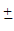byc=0 enclose a
Mathematics Test 11 - Straight Line, Circle - Question 12
The reflection of the point(4,–13) in the line 5x+y+6= 0 is
Mathematics Test 11 - Straight Line, Circle - Question 13
The equation of the bisector of the acute angle between the lines 3x–4y+7 = 0 and 12x+5y–2 = 0 is
Mathematics Test 11 - Straight Line, Circle - Question 14
The vertices of a triangle ABC are (1,1), (4,–2) and (5,5) respectively. Then equation of perpendicular dropped from C to the internal bisector of angle A is
Mathematics Test 11 - Straight Line, Circle - Question 15
A line is such that its segements between the straight lines 5x–y = 4 and 3x+4y–4 = 0 is bisected at the point (1,5). Its equation is
Mathematics Test 11 - Straight Line, Circle - Question 16

The radius of the circle whose centre is on y-axis and which passes through the points (5,2) and (7,–4) is

Mathematics Test 11 - Straight Line, Circle - Question 17
Two circles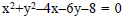and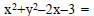0 are such that they
Detailed Solution for Mathematics Test 11 - Straight Line, Circle - Question 17 These are the equation of two circles.The best way to find out whether two circles touches or cuts or do not intersect is to find out the distance between their centres.If this distance is greater than the sum of their radii they don't touch,if equal to sum of radii they just touch, and if less than sum of radii then intersect at two points. If distance is zero then they are concentric.
Mathematics Test 11 - Straight Line, Circle - Question 18
3x+4y–7 = 0 is common tangent at (1,1) to two equal circles of radius 5. Their centres are the points
Mathematics Test 11 - Straight Line, Circle - Question 19

The points (2,3),(0,2),(4,5)and (0,c) are concyclic if the value of c is

Mathematics Test 11 - Straight Line, Circle - Question 20
Equation of the diameter of the circle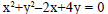which passes through the origin is
Mathematics Test 11 - Straight Line, Circle - Question 21
Which of the following lines is a normal to the circle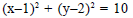Mathematics Test 11 - Straight Line, Circle - Question 22
The equation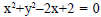represents
Mathematics Test 11 - Straight Line, Circle - Question 23

The coordinates of the point on the circle x2+y2-12x-4y+30=0 which is the farthest from the origin are

Detailed Solution for Mathematics Test 11 - Straight Line, Circle - Question 23
Centre of the given circle is - (-12/2) , - ( -4/2)
This means (6,2) is the centre of the circle.
The farthest point from the origin on the circle has to be the far end of diameter got by joining (0,0) and (6,2)
Equation of line joining these two points is by two point formula
We have x = 3 y
The point of intersection of this line and circle is got by solving these two
We get points (3,1) and (9,3)
Hence required farthest is (9,3)
Mathematics Test 11 - Straight Line, Circle - Question 24
The angle between two tangents from the origin to the circle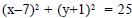is
Mathematics Test 11 - Straight Line, Circle - Question 25
The number of tangents that can be drawn from the point (8,6) to the circle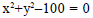is
Mathematics Test 11 - Straight Line, Circle - Question 26
The equation of the common tangent to the circle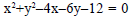,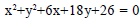at their point of contact is
Mathematics Test 11 - Straight Line, Circle - Question 27
The intercepts made by the circle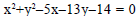on the x-axis and y-axis are respectively
Mathematics Test 11 - Straight Line, Circle - Question 28
The circle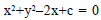touches x-axis, then c =
Mathematics Test 11 - Straight Line, Circle - Question 29
The equation of the passing through the intersection of the circles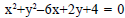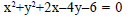and having its centre on the line y = x is
Mathematics Test 11 - Straight Line, Circle - Question 30

The vertex A of a triangle ABC is the point (-2, 3) whereas the line perpendicular to the sides AB and AC are x – y – 4 = 0 and 2x – y – 5 = 0 respectively. The right bisectors of sides meet at P(3/2 , 5/2) . Then the equation of the median of side BC is

## JEE Main & Advanced Mock Test Series

2 videos|325 docs|189 tests
 Use Code STAYHOME200 and get INR 200 additional OFF Use Coupon Code
Information about Mathematics Test 11 - Straight Line, Circle Page
In this test you can find the Exam questions for Mathematics Test 11 - Straight Line, Circle solved & explained in the simplest way possible. Besides giving Questions and answers for Mathematics Test 11 - Straight Line, Circle, EduRev gives you an ample number of Online tests for practice

## JEE Main & Advanced Mock Test Series

2 videos|325 docs|189 tests